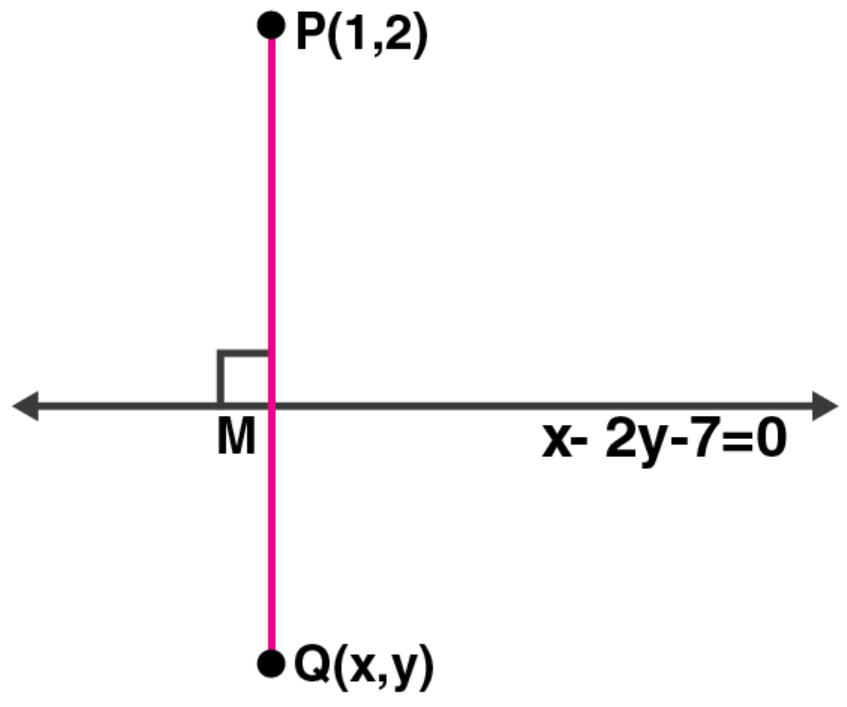Newbie

# Find the image of the point (1, 2) in the line x – 2y – 7 = 0.

• 2

M.L Aggarwal book Important Question of class 10 chapter Based on Equation of a Straight Line for ICSE BOARD.
You have to find the image of the point (1, 2) in the line x – 2y – 7 = 0.
This is the Question Number 40, Exercise 12.2 of M.L Aggarwal.

Share

1.1. Given line equation: x – 2y – 7 = 0 … (i)Draw a perpendicular from point P (1, 2) on the line

Let P’ be the image of P and let its co-ordinates be (x, y)

The slope of the given line is given as,

2y = x – 7

y = (1/2) x – 7

Slope (m1) = ½

Let the slope of line segment PP’ be m2

As PP’ is perpendicular to the given line, product of slopes: m1 x m2 = -1

So, ½ x m2 = -1

m2 = -2

So, the equation of the line perpendicular to the given line and passing through P (1, 2) is

y – 2 = (-2) (x – 1)

y – 2 = -2x + 2

2x + y – 4 = 0 … (ii)

Let the intersection point of lines (i) and (ii) be taken as M.

Solving both the line equations, we have

Multiplying (ii) by 2 and adding with (i)

x – 2y – 7 = 0

4x + 2y – 8 = 0

———————

5x – 15 = 0

x = 15/5 = 3

Putting value of x in (i), we get

3 – 2y – 7 = 0

2y = -4

y = -4/2 = -2

So, the co-ordinates of M are (3, -2)

Hence, its seen that M should be the mid-point of the line segment PP’

(3, -2) = ((x + 1)/2, (y + 2)/2)

(x + 1)/2 = 3

x + 1 = 6

x = 6 – 1 = 5

And,

(y + 2)/2 = -2

y + 2 = -4

y = -4 – 2 = -6

Therefore, the co-ordinates of P’ are (5, -6).

• 4